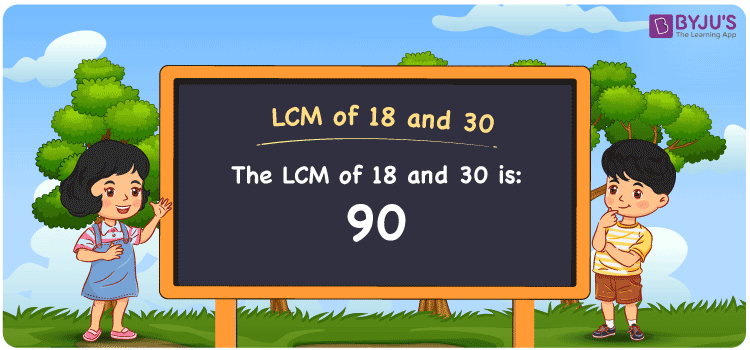Checkout JEE MAINS 2022 Question Paper Analysis : Checkout JEE MAINS 2022 Question Paper Analysis :

# LCM of 18 and 30

LCM of 18 and 30 is 90. Students can learn how to get the least common multiple of 18 and 30 from the common multiples. (18, 36, 54, 72, ….) and (30, 60, 90, 120,….) are the multiples of 18 and 30. The methods used to find the LCM of two numbers are prime factorisation, division and by listing the multiples. The step-wise explanation is provided here for the students’ reference.

## What is LCM of 18 and 30?

The answer to this question is 90. The LCM of 18 and 30 using various methods is shown in this article for your reference. The LCM of two non-zero integers, 18 and 30, is the smallest positive integer 90 which is divisible by both 18 and 30 with no remainder.## How to Find LCM of 18 and 30?

LCM of 18 and 30 can be found using three methods:

• Prime Factorisation
• Division method
• Listing the multiples

### LCM of 18 and 30 Using Prime Factorisation Method

The prime factorisation of 18 and 30, respectively, is given by:

18 = 2 x 3 x 3 = 2¹ x 3²

30 = 2 x 3 x 5 = 2¹ x 3¹ x 5¹

LCM (18, 30) = 90

### LCM of 18 and 30 Using Division Method

We’ll divide the numbers (18, 30) by their prime factors to get the LCM of 18 and 30 using the division method (preferably common). The LCM of 18 and 30 is calculated by multiplying these divisors.

 2 18 30 3 9 15 3 3 5 5 1 5 x 1 1

No further division can be done.

Hence, LCM (18, 30) = 90

### LCM of 18 and 30 Using Listing the Multiples

To calculate the LCM of 18 and 30 by listing out the common multiples, list the multiples as shown below

 Multiples of 18 Multiples of 30 18 30 36 60 54 90 72 120 90 150 108 180

The smallest common multiple of 18 and 30 is 90.

Therefore LCM (18, 30) = 90

## Video Lesson on Applications of LCM## LCM of 18 and 30 Solved Examples

Question: Determine the LCM if the product of two numbers is 540 and the GCD is 6.

Solution:

It is given that,

Product of two numbers = 540

GCD = 6

We know that

LCM x GCD = Product of two numbers

LCM = Product/GCD

LCM = 540/6

LCM = 90

Hence, the LCM is 90.

## Frequently Asked Questions on LCM of 18 and 30

### How to find the LCM of 18 and 30?

We know that the LCM of 18 and 30 is 90. To find the LCM, we should first find the multiples of 18 and 30 and the smallest multiple divisible exactly by 18 and 30 has to be found.

### Write the methods to get the LCM of 18 and 30.

The methods to get the LCM of 18 and 30 are Division Method, Prime Factorization Method and Listing multiples.

### If the LCM of 18 and 30 is 90, what is the GCF?

LCM x GCF = 18 x 30

Given

LCM of 18 and 30 = 90

90 x GCF = 540

GCF = 540/90 = 6